# MAG3110 – Bare metal example project

cancel
Showing results for
Did you mean:

# MAG3110 – Bare metal example project

## MAG3110 – Bare metal example project

Hello community,

This time, I would like to share a simple bare metal example code using the MAG3110 , the digital Magnetometer from NXP.

I created this example code with the FRDM-KL25Z  platform and the sensor expansion board. The complete source code is written in the in collaboration with thetool in order to visualize the magnetic data.

This document guides you through the initialization process and how to appreciate the demonstration.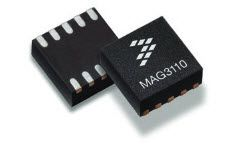Section 1: Initialization of the MKL25Z128 MCU.

Section 2: Initialization of the MAG3110.

Section 3: Simple magnetic hard-iron offset calibration.

Section 4: Output data reading using an interrupt technique.

Section 5: Conversion of the output values.

Section 6: FreeMASTER tool.

## 1. Initialization of the MKL25Z128 MCU

Based on the figure below, the SCL and SDA signals, from the I2C Module, are connected to the PTC1 and PTC2 pins respectively. The INT1 output of the MAG3110 is connected to the PTD4 pin of the KL25Z.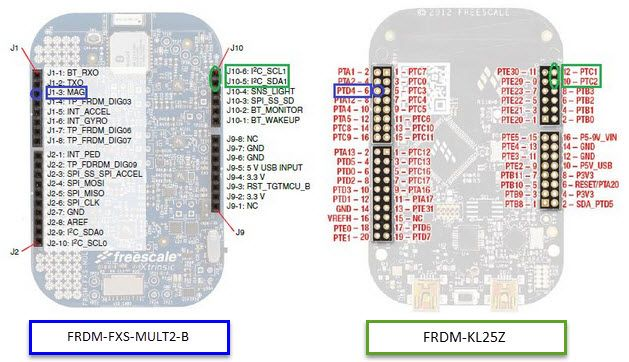Please make sure the 2&3 pins of the J3 are connected together using a jumper at the FRDM-FXS-MULT2-B.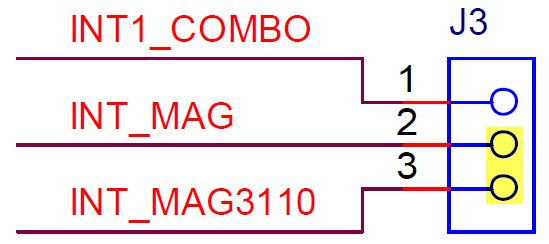The RGB LED from the FRDM-KL25Z is also set using the PTB18 and PTB19 pins as GPIOs.

``````//I2C1 module initialization
SIM_SCGC4 |= SIM_SCGC4_I2C1_MASK;         // Turn on clock to I2C0 module
SIM_SCGC5 |= SIM_SCGC5_PORTC_MASK;        // Turn on clock to Port C module
PORTC_PCR1 = PORT_PCR_MUX(2);             // PTC1 pin is I2C0 SCL line
PORTC_PCR2 = PORT_PCR_MUX(2);             // PTC2 pin is I2C0 SDA line
I2C1_F  = 0x14;                           // SDA hold time = 2.125us, SCL start hold time = 4.25us, SCL stop hold time = 5.125us
I2C1_C1 = I2C_C1_IICEN_MASK;              // Enable I2C0 module

//Configure the PTD4 pin (connected to the INT1 of the MAG3110) for rising edge interrupts
SIM_SCGC5 |= SIM_SCGC5_PORTD_MASK;        // Turn on clock to Port D module
PORTD_PCR4  |= (0|PORT_PCR_ISF_MASK|      // Clear the interrupt flag
PORT_PCR_MUX(0x1)|      // PTD4 is configured as GPIO
PORT_PCR_IRQC(0x09));   // PTD4 is configured for rising edge interrupts (MAG3110 generates low to high signal)

//Configure RGB LED
SIM_SCGC5 |= SIM_SCGC5_PORTB_MASK;        // Turn on clock to Port B module

PORTB_PCR19 = PORT_PCR_MUX(1);            // PTB19 is configured as GPIO
GPIOB_PDDR |= (1 << 19);                  // Configure pin as output
GPIOB_PSOR |= (1 << 19);                  // Turn OFF GREEN LED

PORTB_PCR18 = PORT_PCR_MUX(1);            // PTB18 is configured as GPIO
GPIOB_PDDR |= (1 << 18);                  // Configure pin as output
GPIOB_PCOR |= (1 << 18);                  // Turn ON RED LED

//Enable PORTD interrupt on NVIC
NVIC_EnableIRQ(PORTD_IRQn);               // Enable interrupts
NVIC_ClearPendingIRQ(PORTD_IRQn);         // Clear pending interrupts
‍‍‍‍‍‍‍‍‍‍‍‍‍‍‍‍‍‍‍‍‍‍‍‍‍‍‍‍‍‍‍‍‍‍‍‍‍‍‍‍‍‍‍‍‍‍‍‍‍‍‍‍‍‍‍‍‍‍‍‍‍``````

## 2. Initialization of the MAG3110

The MAG3110 is capable of measuring magnetic fields with an output data rate (ODR) up to 80 Hz. In this case, the ODR of the MAG3110 is set at 20Hz.

The automatic resets are enabled. When new measurement data is available, the INT1 pin triggers a software interrupt.

The WHO_AM_I register is read in order to verify the correct communication with the magnetometer.

``````I2C_WriteRegister(MAG3110_I2C_ADDRESS, CTRL_REG2, 0x80);  // Enable automatic resets

I2C_WriteRegister(MAG3110_I2C_ADDRESS, CTRL_REG1, 0x11);  // ODR 20Hz (0.05s), Active mode

‍‍‍‍‍‍‍‍‍‍‍‍‍‍‍``````

## 3. Simple magnetic hard-iron offset calibration.

Please note that the magnetometer readings must be corrected for Hard-Iron and Soft-Iron effects. If you are interested in more complex algorithms you may refer to the NXP E-Compass Software.

As an alternative, a method to calibrate the hard iron offset is rotating the MAG3110 in a figure of eight twisting motions for a few seconds, record the minimum and maximum magnetometer readings, compute the corresponding offset values by using the min/max averaging and then either subtract these offset values from the current magnetometer measurements or write them in the user offset registers with CTRL_REG2[RAW] = 0.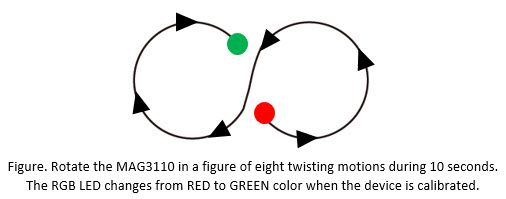The hard iron offset calibration is done as follow:

``````short Xout_16_bit_avg, Yout_16_bit_avg, Zout_16_bit_avg;
short Xout_16_bit_max, Yout_16_bit_max, Zout_16_bit_max;
short Xout_16_bit_min, Yout_16_bit_min, Zout_16_bit_min;
short i=0;

while (i < 200) // Calibration process ~10s (200 samples * 1/20Hz)
{
{

Xout_16_bit = ((short) (MagData<<8 | MagData));        // Compute 16-bit X-axis output value
Yout_16_bit = ((short) (MagData<<8 | MagData));        // Compute 16-bit Y-axis output value
Zout_16_bit = ((short) (MagData<<8 | MagData));        // Compute 16-bit Z-axis output value

if (i == 0)
{
Xout_16_bit_max = Xout_16_bit;
Xout_16_bit_min = Xout_16_bit;

Yout_16_bit_max = Yout_16_bit;
Yout_16_bit_min = Yout_16_bit;

Zout_16_bit_max = Zout_16_bit;
Zout_16_bit_min = Zout_16_bit;
}

// Check to see if current sample is the maximum or minimum X-axis value
if (Xout_16_bit > Xout_16_bit_max) {Xout_16_bit_max = Xout_16_bit;}
if (Xout_16_bit < Xout_16_bit_min) {Xout_16_bit_min = Xout_16_bit;}

// Check to see if current sample is the maximum or minimum X-axis value
if (Yout_16_bit > Yout_16_bit_max) {Yout_16_bit_max = Yout_16_bit;}
if (Yout_16_bit < Yout_16_bit_min) {Yout_16_bit_min = Yout_16_bit;}

// Check to see if current sample is the maximum or minimum X-axis value
if (Zout_16_bit > Zout_16_bit_max) {Zout_16_bit_max = Zout_16_bit;}
if (Zout_16_bit < Zout_16_bit_min) {Zout_16_bit_min = Zout_16_bit;}

i++;
}
}

Xout_16_bit_avg = (Xout_16_bit_max + Xout_16_bit_min) / 2;    // X-axis hard-iron offset
Yout_16_bit_avg = (Yout_16_bit_max + Yout_16_bit_min) / 2;    // Y-axis hard-iron offset
Zout_16_bit_avg = (Zout_16_bit_max + Zout_16_bit_min) / 2;    // Z-axis hard-iron offset

// Left-shift by one as magnetometer offset registers are 15-bit only, left justified
Xout_16_bit_avg <<= 1;
Yout_16_bit_avg <<= 1;
Zout_16_bit_avg <<= 1;

I2C_WriteRegister(MAG3110_I2C_ADDRESS, CTRL_REG1, 0x00);  // Standby mode

// Set Offset
I2C_WriteRegister(MAG3110_I2C_ADDRESS, OFF_X_MSB, (char)((Xout_16_bit_avg >>8) & 0xFF));
I2C_WriteRegister(MAG3110_I2C_ADDRESS, OFF_Y_MSB, (char)((Yout_16_bit_avg >>8) & 0xFF));
I2C_WriteRegister(MAG3110_I2C_ADDRESS, OFF_Z_MSB, (char)((Zout_16_bit_avg >>8) & 0xFF));

I2C_WriteRegister(MAG3110_I2C_ADDRESS, CTRL_REG1, 0x11);  //  Active mode again
‍‍‍‍‍‍‍‍‍‍‍‍‍‍‍‍‍‍‍‍‍‍‍‍‍‍‍‍‍‍‍‍‍‍‍‍‍‍‍‍‍‍‍‍‍‍‍‍‍‍‍‍‍‍‍‍‍‍‍‍‍‍‍‍‍‍‍‍‍‍‍‍‍‍‍‍‍‍‍‍‍‍‍‍‍‍‍‍‍‍‍‍‍‍‍‍‍‍‍‍‍‍‍‍‍‍‍‍‍‍‍‍‍‍‍‍‍‍‍‍‍‍‍‍‍‍‍‍‍‍‍‍‍``````

## 4. Output data reading using an interrupt technique.

At the ISR, the interrupt flag is clear and the DataReady variable is set in order to know that a new magnetic measurement is ready.

``````void PORTD_IRQHandler()
{
PORTD_PCR4 |= PORT_PCR_ISF_MASK;            // Clear the interrupt flag
}
‍‍‍‍‍‍‍‍‍‍‍``````

## 5. Conversion of the output values

The output values from magnetometer are converted to signed 16-bit integer values and afterwards to real values in microtesla (µT).

``````for(;;){

{

// 16-bit magnetometer data
Xout_16_bit = ((short) (MagData<<8 | MagData));        // Compute 16-bit X-axis output value
Yout_16_bit = ((short) (MagData<<8 | MagData));        // Compute 16-bit Y-axis output value
Zout_16_bit = ((short) (MagData<<8 | MagData));        // Compute 16-bit Z-axis output value

// Magnetometer data converted to microteslas
Xout_uT = (float)Xout_16_bit / SENSITIVITY;     // Compute X-axis output magnetic value in uT
Yout_uT = (float)Yout_16_bit / SENSITIVITY;     // Compute Y-axis output magnetic value in uT
Zout_uT = (float)Zout_16_bit / SENSITIVITY;     // Compute Z-axis output magnetic value in uT
}
}‍‍‍‍‍‍‍‍‍‍‍‍‍‍‍‍‍‍‍‍‍‍‍‍‍‍‍‍‍‍‍‍‍‍‍‍‍‍‍‍‍‍‍``````

## 6. FreeMASTER

FreeMASTER is a user-friendly real-time debug monitor and data visualization tool that you can use for any application development and information management. In this case, it is used in order to visualize the magnetic data.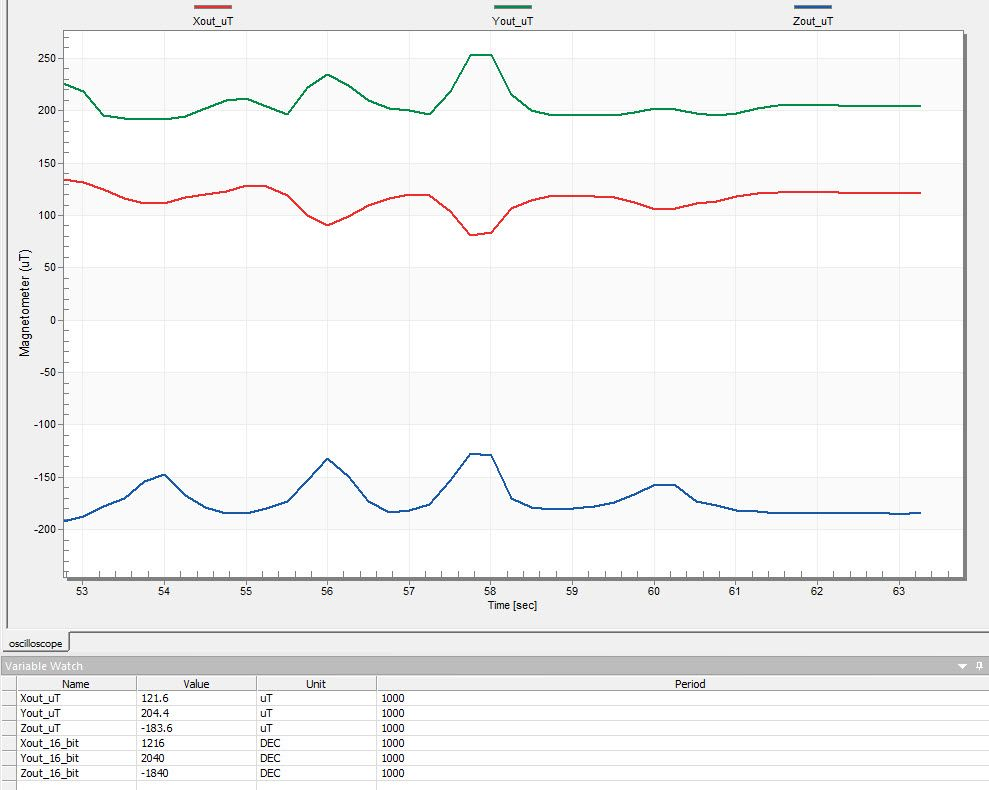In case you have problems with the communication port, please go to Project / Options…

Select Plug-in Module and choose the FreeMASTER BDM Communication Plug-in option.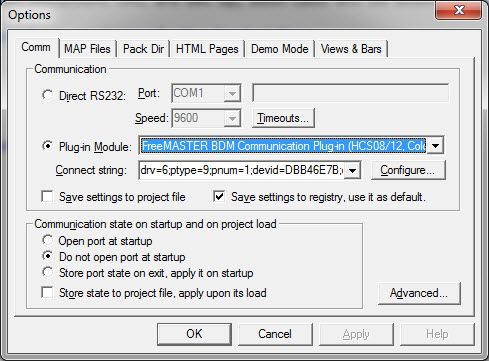Select configure and make sure the P&E Kinetis is selected.

Select OK and Start the communication.

Please find attached the complete source code, including the FreeMASTER project.

You are invited to take part of the NXP community where you can post all your questions and you may find useful material for your projects.

I hope you find useful and funny this sample project. Any suggestion will be appreciated.Best Regards,

David

Labels (1)
• ### Magnetic Sensors

Version history
Revision #:
2 of 2
Last update:
‎09-10-2020 01:39 AM
Updated by:Contributors